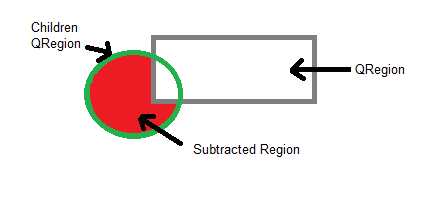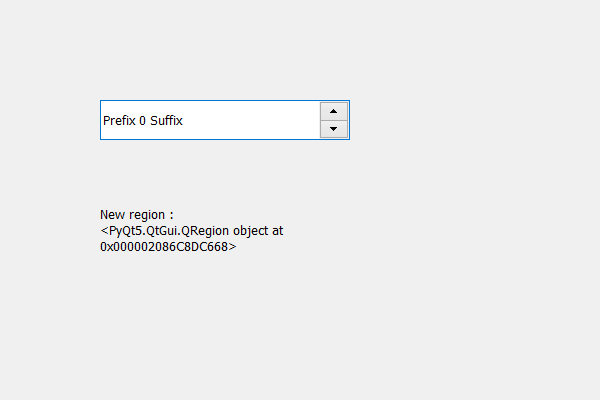Related Articles

# PyQt5 QSpinBox – Subtracting region from Children Region

• Last Updated : 19 May, 2020

In this article we will see how we can subtract the region from children region of spin box, children region holds the combined region occupied by the spin box’s children. In order to get the children region we use `childrenRegion` method. Below is the representation of how subtracted region looks like.In order to do this we use subtracted method with the children region object of the spin box

Syntax : children_region.subtracted(region)

Argument : It takes QRegion object as argument

Return : It returns None

Below is the implementation

 `# importing libraries``from` `PyQt5.QtWidgets ``import` `*` `from` `PyQt5 ``import` `QtCore, QtGui``from` `PyQt5.QtGui ``import` `*` `from` `PyQt5.QtCore ``import` `*` `import` `sys`` ` ` ` `class` `Window(QMainWindow):`` ` `    ``def` `__init__(``self``):``        ``super``().__init__()`` ` `        ``# setting title``        ``self``.setWindowTitle(``"Python "``)`` ` `        ``# setting geometry``        ``self``.setGeometry(``100``, ``100``, ``600``, ``400``)`` ` `        ``# calling method``        ``self``.UiComponents()`` ` `        ``# showing all the widgets``        ``self``.show()`` ` `        ``# method for widgets`` ` `    ``def` `UiComponents(``self``):``        ``# creating spin box``        ``self``.spin ``=` `QSpinBox(``self``)`` ` `        ``# setting geometry to spin box``        ``self``.spin.setGeometry(``100``, ``100``, ``250``, ``40``)`` ` `        ``# setting range to the spin box``        ``self``.spin.setRange(``0``, ``999999``)`` ` `        ``# setting prefix to spin``        ``self``.spin.setPrefix(``"Prefix "``)`` ` `        ``# setting suffix to spin``        ``self``.spin.setSuffix(``" Suffix"``)`` ` `        ``# getting the children region``        ``children_region ``=` `self``.spin.childrenRegion()`` ` `        ``# creating a label``        ``label ``=` `QLabel(``self``)``        ``label.setWordWrap(``True``)`` ` `        ``# setting geometry to the label``        ``label.setGeometry(``100``, ``200``, ``200``, ``60``)`` ` `        ``# creating a region``        ``region ``=` `QRegion(QRect(``1``, ``1``, ``20``, ``20``))`` ` `        ``# subtracting a region``        ``children_region.subtracted(region)`` ` `        ``# setting text to the label``        ``label.setText(``"New region : "` `+` `str``(children_region))`` ` ` ` `# create pyqt5 app``App ``=` `QApplication(sys.argv)`` ` `# create the instance of our Window``window ``=` `Window()``window.spin.setFocus()``# start the app``sys.exit(App.``exec``())`

Output :Attention geek! Strengthen your foundations with the Python Programming Foundation Course and learn the basics.

To begin with, your interview preparations Enhance your Data Structures concepts with the Python DS Course. And to begin with your Machine Learning Journey, join the Machine Learning – Basic Level Course

My Personal Notes arrow_drop_up